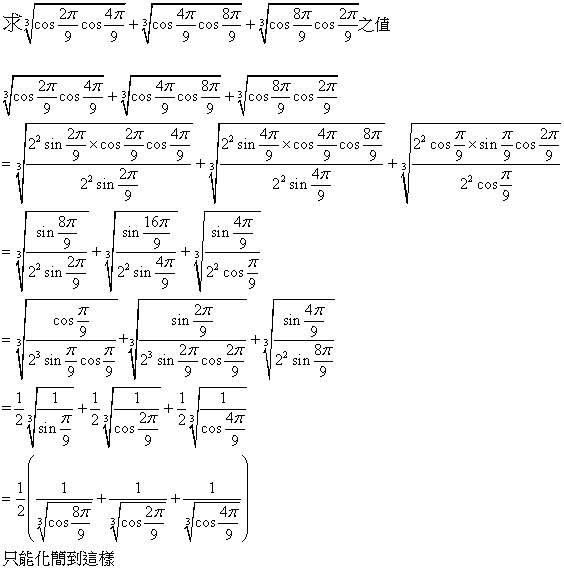﻿ Math Pro 數學補給站$$\displaystyle \theta=\frac{2 \pi}{9},\frac{4 \pi}{9},\frac{8 \pi}{9},$$滿足$$cos 3\theta=-\frac{1}{2}$$
$$cos3 \theta=4cos^3 \theta-3cos \theta$$

$$x(8x^2-6)=-1$$　，　$$x^2(8x^2-6)^2=1$$　，　$$y(8y-6)^2=1$$　，　$$64y^3-96y^2+36y-1=0$$

$$8x^3+1=6x$$　，　$$(8x^3+1)^3=(6x)^3$$　，　$$(8y+1)^3=216y$$　，　$$512y^3+192y^2-192y+1=0$$

$$8x^3+1=6x$$　，　$$\root 3 \of {8x^3+1}=\root 3 \of {6x}$$Jiří Herman, Radan Kučera, Jaromír Šimša
Equations and Inequalities:Elementary Problems and Theorems in Algebra and Number Theory

$$\displaystyle \root 3 \of {cos \frac{2 \pi}{9}}+\root 3 \of{cos \frac{4 \pi}{9}}+\root 3 \of{cos \frac{8 \pi}{9}}=\root 3 \of{\frac{3}{2}\root 3 \of 9-3}$$
$$\displaystyle \root 3 \of{cos \frac{2 \pi}{9}cos \frac{4 \pi}{9}}+\root 3 \of{cos \frac{4 \pi}{9}cos \frac{8 \pi}{9}}+\root 3 \of{cos \frac{8 \pi}{9}cos \frac{2 \pi}{9}}=\root 3 \of{\frac{3}{4}(1-\root 3 \of 9)}$$

109.7.12補充
$$\root 3\of{cos40^{\circ}}+\root 3\of{cos80^{\circ}}-\root 3\of{cos20^{\circ}}=\root 3\of\frac{3}{2}(\root 3 \of{9}-2)$$

$$\displaystyle\left\{\begin{array}{ccc}\sqrt{y_1}+\sqrt{y_2}+\sqrt{y_3}&=&A\\ \sqrt{y_1y_2}+\sqrt{y_2y_3}+\sqrt{y_3y_1}&=&B\\ \sqrt{y_1y_2y_3}&=&C\end{array}\right.$$

（ｉ）將 $$m,p,q$$ 分別以 $$\displaystyle\sqrt{y_1},\sqrt{y_2},\sqrt{y_3}$$ 帶入※※，

$$\displaystyle\Rightarrow A^3=3AB+\frac{3}{2}$$　．．．．（第一式）

（ｉｉ）將 $$m,p,q$$ 分別以 $$\sqrt{y_1y_2},\sqrt{y_2y_3},\sqrt{y_3y_1}$$ 帶入※※，

$$\displaystyle\Rightarrow B^3=-\frac{3}{2}AB-\frac{3}{2}$$　‧‧‧‧（第二式）

$$\displaystyle \root 3 \of {\cos \frac{2 \pi}{9}}+\root 3 \of{\cos \frac{4 \pi}{9}}+\root 3 \of{\cos \frac{8 \pi}{9}}=\root 3 \of{\frac{3}{2}\root 3 \of 9-3}$$

$$\displaystyle \root 3 \of{\cos \frac{2 \pi}{9}\cos \frac{4 \pi}{9}}+\root 3 \of{\cos \frac{4 \pi}{9}\cos \frac{8 \pi}{9}}+\root 3 \of{\cos \frac{8 \pi}{9}\cos \frac{2 \pi}{9}}=\root 3 \of{\frac{3}{4}(1-\root 3 \of 9)}$$

^____^

Prove the identity
$$\displaystyle \root 3 \of {cos \frac{2 \pi}{7}}+\root 3 \of{cos \frac{4 \pi}{7}}+\root 3 \of{cos \frac{8 \pi}{7}}=\root 3 \of{\frac{1}{2}(5-3\root 3 \of 7)}$$

https://artofproblemsolving.com/community/c6h370998
https://artofproblemsolving.com/community/c6h363946p1998666
https://artofproblemsolving.com/community/c6h1079891p4739612

$$\displaystyle \omega=cos \frac{2 \pi}{7}+i sin \frac{2 \pi}{7}$$
$$\displaystyle \alpha=\omega+\omega^6=2 cos \frac{2 \pi}{7}$$，
$$\displaystyle \beta=\omega^2+\omega^5=2 cos \frac{4 \pi}{7}$$，
$$\displaystyle \gamma=\omega^3+\omega^4=2 cos \frac{6 \pi}{7}$$，

(88高中數學能力競賽　宜花東區試題)
h ttp://www.math.nuk.edu.tw/senpengeu/HighSchool/2000_Taiwan_High_Ilan_02.pdf連結已失效

(88高中數學能力競賽　高屏區試題)
h ttp://www.math.nuk.edu.tw/senpengeu/HighSchool/2000_Taiwan_High_Pingtung_02.pdf連結已失效

－－－－－－－－－－－－－－－－－－－－－－－－－－－－－－
Prove that the number $$\displaystyle c=\root 3 \of {\frac{1}{9}}+\root 3 \of {-\frac{2}{9}}+\root 3 \of {\frac{4}{9}}$$ is a zero of $$F(x)=x^3+\root 3 \of 6 x^2-1$$.

(1995日本奧林匹克預選賽)

(92高中數學能力競賽，高中數學101　P25)

110.9.23補充
$$\displaystyle \root 3 \of{\root 3 \of 2 -1}=\root 3 \of a+\root 3 \of b+\root 3 \of c$$，其中$$a$$、$$b$$、$$c \in Q$$。求$$a+b+c=$$

(109第1學期中山大學雙週一題)

110.2.25補充

111.1.10補充

－－－－－－－－－－－－－－－－－－－－－－－－－－－－－－
For the expression
$$\displaystyle Q(n)=\frac{1}{2}\cdot \frac{3}{4}\cdot \frac{5}{6}\cdot \cdot \cdot \frac{2n-1}{2n}$$　　　　　　　　( $$n \ge 2$$ )
we will prove the bounds $$\displaystyle \frac{1}{2 \sqrt{n}}<Q(n)<\frac{1}{\sqrt{2n+1}}$$.

$$\displaystyle a_n=\frac{1 \cdot 3 \cdot 5 \cdot \cdot \cdot (2n-1)}{2 \cdot 4 \cdot 6 \cdot \cdot \cdot (2n)}$$，試求$$\displaystyle \lim_{n \to \infty}a_n$$。

－－－－－－－－－－－－－－－－－－－－－－－－－－－－－－
Show that for arbitary $$x,y,z \in R$$ we have
$$\displaystyle (\frac{x}{2}+\frac{y}{3}+\frac{z}{6})^2 \le \frac{x^2}{2}+\frac{y^2}{3}+\frac{z^2}{6}$$　　　　　　　　(43)
Furthermore,determine when equality occures in (43).

(90高中數學能力競賽　中彰投區試題)
h ttp://www.math.nuk.edu.tw/senpengeu/HighSchool/2002_Taiwan_High_Taichung_02.pdf　連結已失效

－－－－－－－－－－－－－－－－－－－－－－－－－－－－－－
Show that the inequality $$\sqrt{x+1}+\sqrt{2x-3}+\sqrt{50-3x}\le 12$$ holds for all values $$x \in R$$ for which the left-hand side is defined.

SOLUTION.　By (48) with $$n=3$$, $$u_1=\sqrt{x+1}$$,$$u_2=\sqrt{2x-3}$$, and $$u_3=\sqrt{50-3x}$$
we have $$(\sqrt{x+1}+\sqrt{2x-3}+\sqrt{50-3x})^2 \le 3(x+1+2x-3+50-3x)=144$$,
from which, upon taking the square root, we obtain the result.

(2009大陸高中數學競賽)

－－－－－－－－－－－－－－－－－－－－－－－－－－－－－－

Given an aribitrary prime p, solve the Diophantine equation $$x^4+4^x=p$$.(第245頁)
SOLUTION.　For any integer $$x<0$$, $$4^x$$ is not an integer, and neither is $$x^4+4^x$$.
Thus for any prime p, the given equation has no negative solution.
For $$x=0$$ we have $$0^4+4^0=1$$, which is not a prime, and for $$x=1$$ we have $$1^4+4^1=5$$, a prime.
We will now show that for any integer $$x\ge 2$$, the number $$x^4+4^x$$ is composite. If $$x=2k$$ is even, where $$k \in N$$, then
$$x^4+4^x=2^4 k^4+4^{2k}=16(k^4+4^{2(k-1)})$$,
which is a composite number. If $$x=2k+1$$( $$k \in N$$ ) is odd, then
$$x^4+4^x=x^4+4 \cdot 4^{2k}=[x^4+4x^2(2^k)^2+4(2^k)^4]-4x^2(2^k)^2$$
$$=[x^2+2(2^k)^2]^2-(2x \cdot 2^k)^2$$
$$=[x^2+2x \cdot 2^k+2(2^k)^2][x^2-2x \cdot 2^k+2(2^k)^2]$$
$$=[(x+2^k)^2+2^{2k}][(x-2^k)^2+2^{2k}]$$,
which is again compostie, since $$(x±2^k)^2+2^{2k}\ge 2^{2k} \ge 2^2 >1$$.
To summarize, for $$p=5$$ the given equation has the unique solution $$x=1$$,while there are no solutions for any prime $$p \ne 5$$

Prove that if $$n>1$$ then $$n^4+4^n$$ is compostie.
http://www.artofproblemsolving.com/Forum/viewtopic.php?t=328143

(藍藍天上一朵雲　歷屆教甄考題整理2005版第75題)

Prove that there are infinitely many natural numbers a with the following property: the number $$z=n^4+a$$ is not prime for any natural number n.
(1969IMO，http://www.artofproblemsolving.c ... p/1969_IMO_Problems)

Compute $$\displaystyle \frac{(10^4+324)(22^4+324)(34^4+324)(46^4+324)(58^4+324)}{(4^4+324)(16^4+324)(28^4+324)(40^4+324)(52^4+324)}$$.
(1987AIME)
101.1.10補充

(88高中數學能力競賽　宜花東區試題)這題

\begin{align} & {{\omega }^{7}}=1,\omega \ne 1 \\ & {{\omega }^{6}}+{{\omega }^{5}}+{{\omega }^{4}}+{{\omega }^{3}}+{{\omega }^{2}}+\omega =-1 \\ & \\ & \alpha +\beta +\gamma =\omega +{{\omega }^{6}}+{{\omega }^{2}}+{{\omega }^{5}}+{{\omega }^{3}}+{{\omega }^{4}}=-1 \\ & \alpha \beta +\beta \gamma +\gamma \alpha =\left( \omega +{{\omega }^{6}} \right)\left( {{\omega }^{2}}+{{\omega }^{5}} \right)+\left( {{\omega }^{2}}+{{\omega }^{5}} \right)\left( {{\omega }^{3}}+{{\omega }^{4}} \right)+\left( {{\omega }^{3}}+{{\omega }^{4}} \right)\left( \omega +{{\omega }^{6}} \right) \\ & =2\left( {{\omega }^{6}}+{{\omega }^{5}}+{{\omega }^{4}}+{{\omega }^{3}}+{{\omega }^{2}}+\omega \right)=-2 \\ & \alpha \beta \gamma =\left( \omega +{{\omega }^{6}} \right)\left( {{\omega }^{2}}+{{\omega }^{5}} \right)\left( {{\omega }^{3}}+{{\omega }^{4}} \right)=2+\left( {{\omega }^{6}}+{{\omega }^{5}}+{{\omega }^{4}}+{{\omega }^{3}}+{{\omega }^{2}}+\omega \right)=1 \\ \end{align}

 歡迎光臨 Math Pro 數學補給站 (https://math.pro/db/) 論壇程式使用 Discuz! 6.1.0